Interactive Lecture Demonstration

Prediction Sheet—Vectors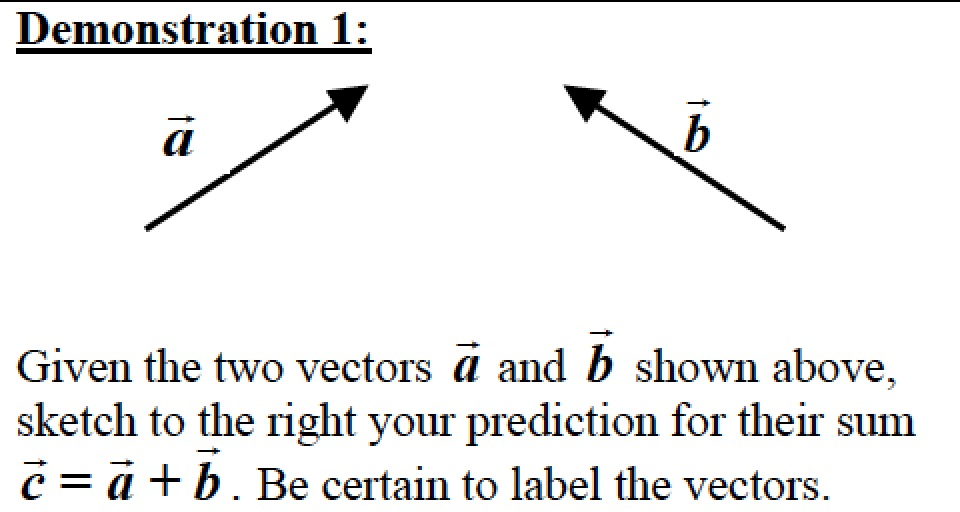Only after you have sketched your prediction, open the Vector Addition simulation: https://phet.colorado.edu/sims/html/vector-addition/latest/vector-addition_en.html and open Explore 2D. Drag the two vectors anywhere on the graph grid, adjust their lengths carefully, and then click on the Sum---> box to view the vector sum.  Does this agree with your prediction?   Move the vectors around to show how the Triangle Rule results in the vector sum. Describe how this results in the correct sum.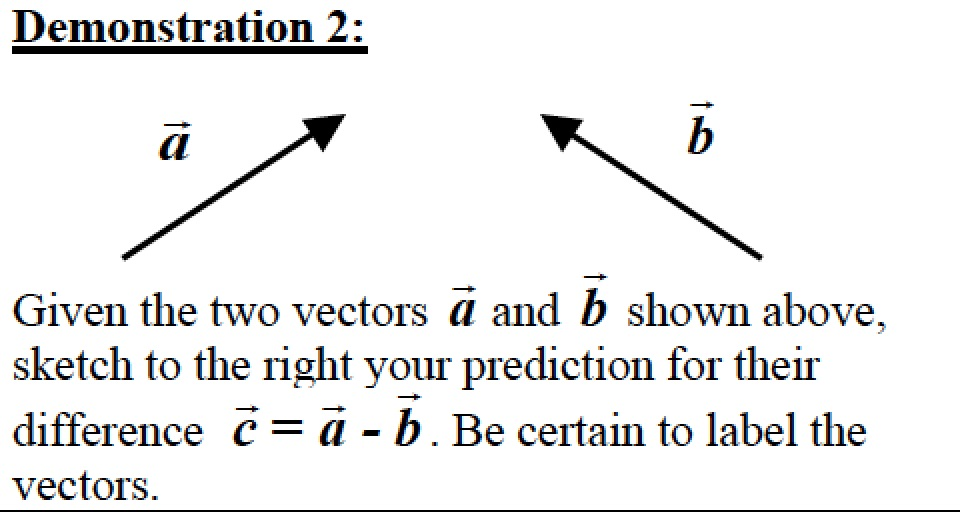Only after you have sketched your prediction, use the Vector Addition simulation. Recall that the vector difference a - b is the same as the sum of a + (-b). Does the result agree with your prediction? If not, explain why.   Move the vectors around to show how the Triangle Rule results in the vector difference. Describe how this results in the correct difference.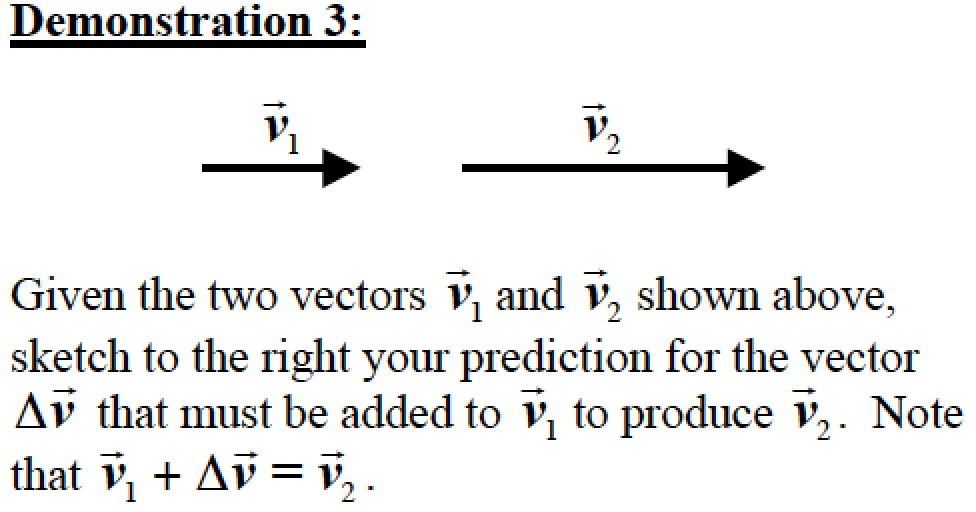Only after you have sketched your prediction, again use the Vector Addition simulation. Use the vectors a and b for the two v vectors and recall that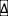v = v2 - v1. Does the result agree with your prediction? If not, explain why.   Also use the simulation to verify that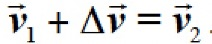.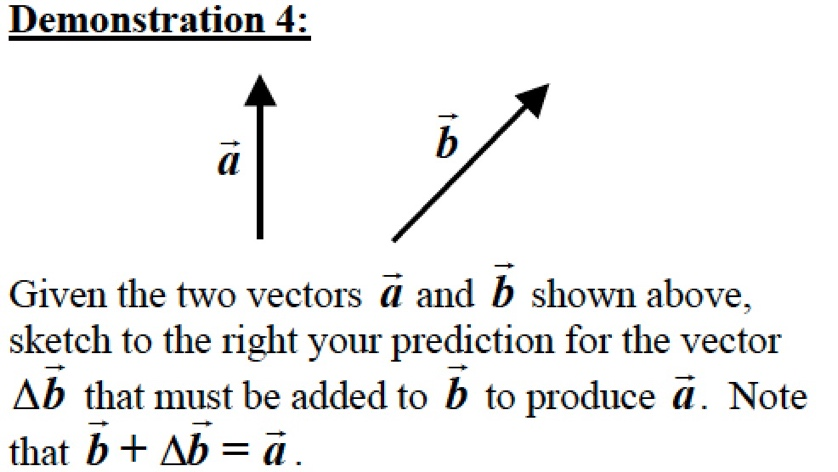Only after you have sketched your prediction, again use the Vector Addition simulation. Recall thatb = a - b. Does the result agree with your prediction? If not, explain why.   Also use the simulation to verify that b +b = a.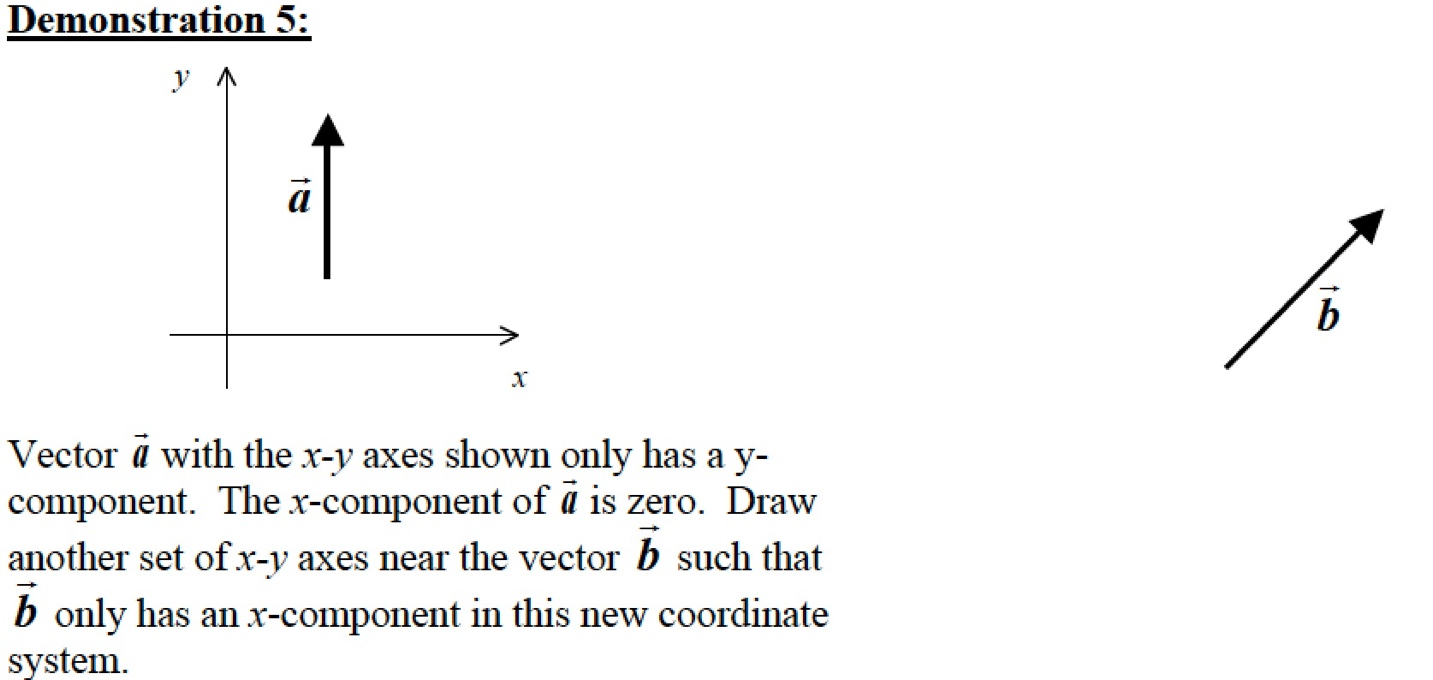Only after you have sketched your prediction, click here to see the result. Compare the result to your prediction and explain any differences.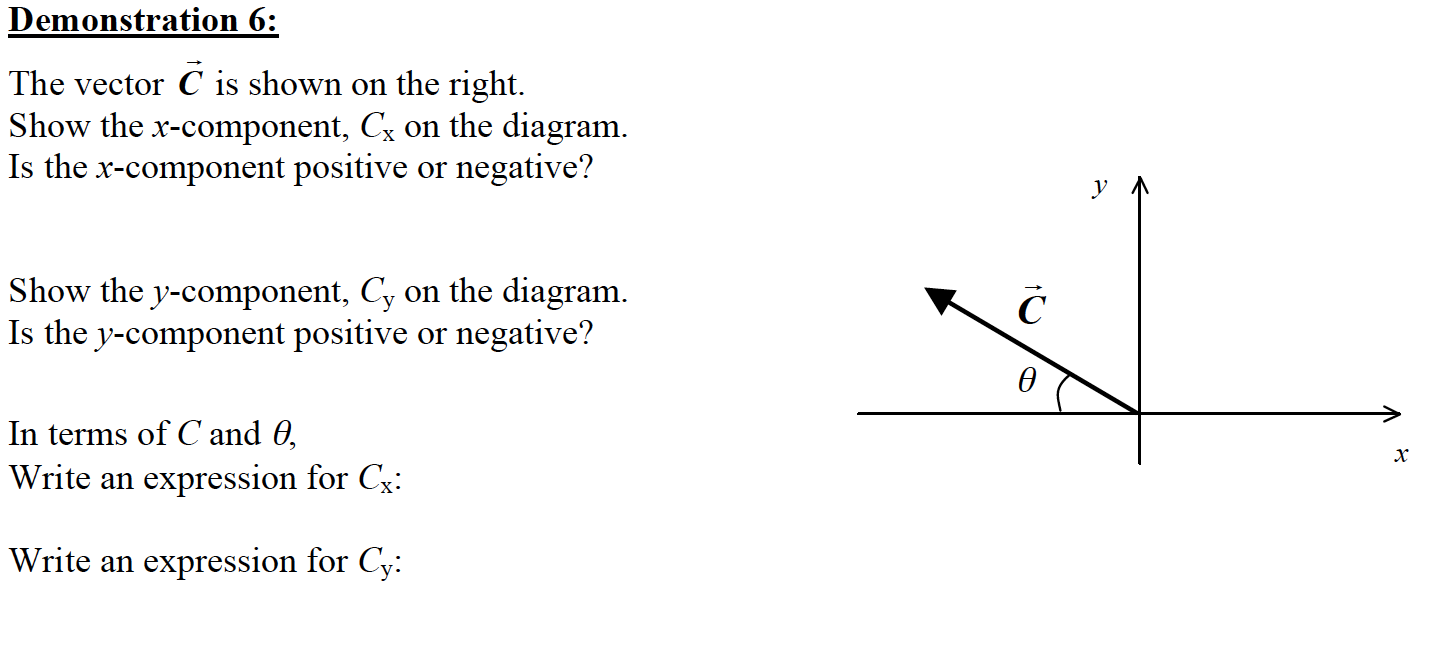Only after you have sketched your prediction, click here to see the result. Compare the result to your prediction and explain any differences.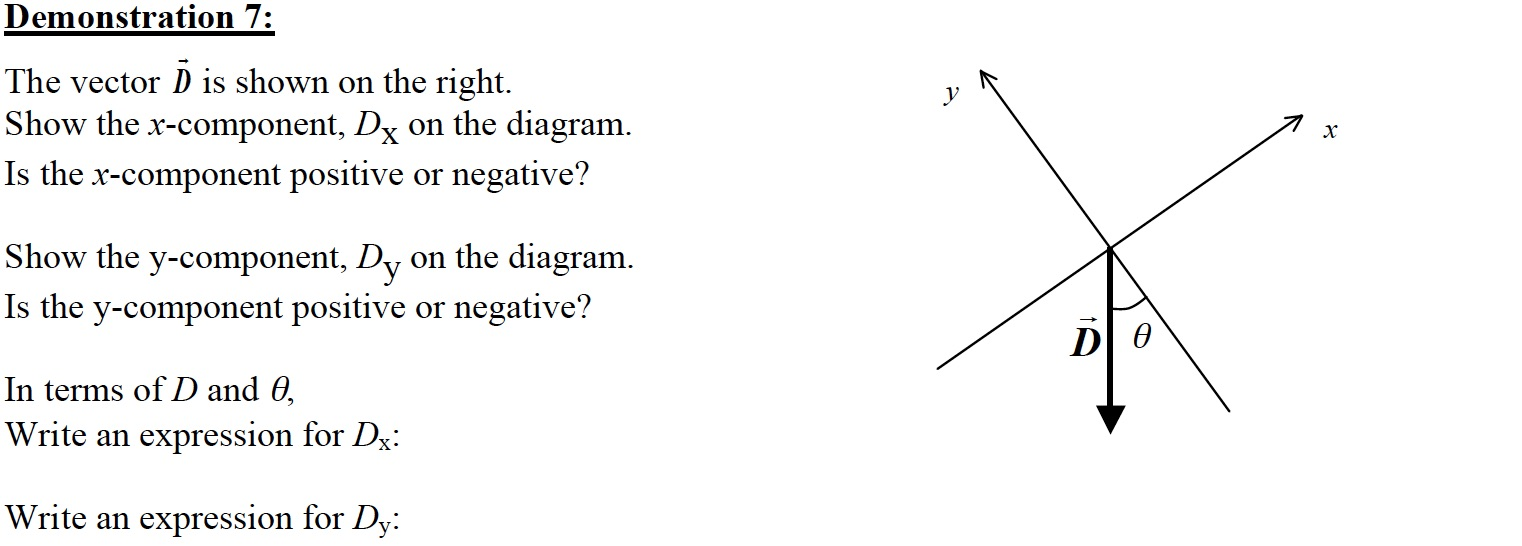Only after you have sketched your prediction, click here to see the result. Compare the result to your prediction and explain any differences.